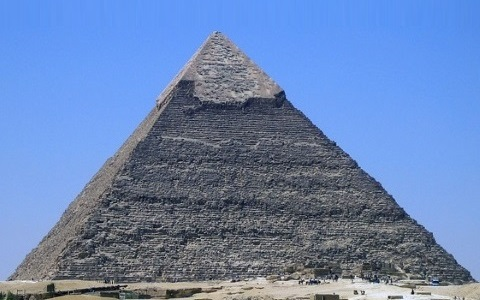#### You may also like### Summing Consecutive Numbers

15 = 7 + 8 and 10 = 1 + 2 + 3 + 4. Can you say which numbers can be expressed as the sum of two or more consecutive integers?### Always the Same

Arrange the numbers 1 to 16 into a 4 by 4 array. Choose a number. Cross out the numbers on the same row and column. Repeat this process. Add up you four numbers. Why do they always add up to 34?### Fibs

The well known Fibonacci sequence is 1 ,1, 2, 3, 5, 8, 13, 21.... How many Fibonacci sequences can you find containing the number 196 as one of the terms?

# Number Pyramids

##### Age 11 to 14Challenge Level

In a number pyramid, the numbers on the lower layers determine the numbers above them.

Start by choosing three single-digit numbers and enter them in the bottom row of the interactive number pyramid.Try entering some different numbers in the bottom row - you don't have to stick to single-digit numbers.
Can you work out how the numbers in the upper layers are generated?

Here are some questions to consider:

If I tell you the numbers on the bottom layer, can you work out the top number without working out the middle layer?

If you change the order of the numbers on the bottom layer, will the top number change?

Given any three numbers for the bottom, how can you work out the largest possible number that could go at the top?

If I give you a target for the top number, can you quickly find three possible numbers for the bottom?

Can you adapt what you discovered about 3-layer pyramids to larger pyramids?

Here is an interactive number pyramid with four layers so you can test out your ideas.

You might now like to have a go at More Number Pyramids